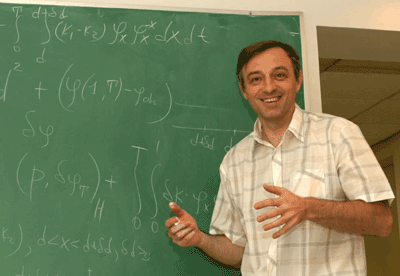Dr. Pavel B. Dubovski
E-mail: pdubovsk "at" stevens.edu
Office: Kidde 226Research Interests
Publications
Teaching

 Research interests:

Applied Analysis, Kinetic Equations and Conservation Laws, Integral Equations, Mathematical Modeling, Inverse Problems.

 Teaching:

Ma 221. Differential Equations (spring 2011)
Ma 116. Calculus II (fall 2010)
Ma 116. Calculus II (fall 2009)
Ma 650. Partial Differential Equations (fall 2009)
Mathematical circle

 Previous Teaching:
Ma 116. Calculus II (fall 2008)
Ma 650. Partial Differential Equations (fall 2008)

MA 134. Discrete Mathematics (summer 2008)
MA 221. Differential Equations (summer 2008)
MA 134. Discrete Mathematics (spring 2008)
MA 649. Intermediate Differential Equations (spring 2008)
MA 116. Calculus II (fall 2007)
MA 650. Partial Differential Equations (fall 2007)
MA 116. Calculus II (fall 2006)
MA 635. Real Analysis (fall 2006)
MA 134. Discrete Mathematics (summer 2006)
MA 116. Calculus II (fall 2004)

 Some publications (of 50).

- "Problem Solution in Mathematical Physics," (textbook). Moscow: Nauka, 2002 (with V.I.Agoshkov and V.P.Shutyaev).
- "Mathematical Theory of Coagulation," (monograph). Seoul: Seoul National University, 1994.
- "Reconstruction of the Source Function for the Coagulation-Fragmentation Equation," Russian J. Numerical Analysis and Mathematical Modeling 17, No. 4, 2002 (with V.I.Agoshkov)
- "Solubility of the transport equation in the kinetics of coagulation and fragmentation," Izvestiya: Mathematics 65, No. 1, 1-22, 2001.
- "Analytic solution of the fragmentation equation by means of the adjoint equations methods," Differential Equations 36, No. 10, 1385-1392 (2000)
- "Cancer as a catastrophe of strange attractor," Regional Projects in Natural Sciences 4. Kaluga: Eidos, 2003 (with R.V.Plykin, E.A.Sataev, E.O.Fedorovskaya, in Russian).
- "Functional and measure-valued solutions of the Euler equations for flows of incompressible fluids," Arch. Rational Mech. Anal. 129, 1995, 385-396 (with D. Chae)
- "A "triangle" of interconnected coagulation models," J. Phys. A: Math. Gen. 32, No.5, 1999, 781-793.
- "Exact solutions for the coagulation-fragmentation equation," J. Phys. A: Math. Gen. 25, 1992, 4737-4744 (with V.Galkin and I.Stewart)
- "Nuclear Reactor." Patent of Russia No. 2017242 of 30 July 1994.

Last update: May 19, 2006 >